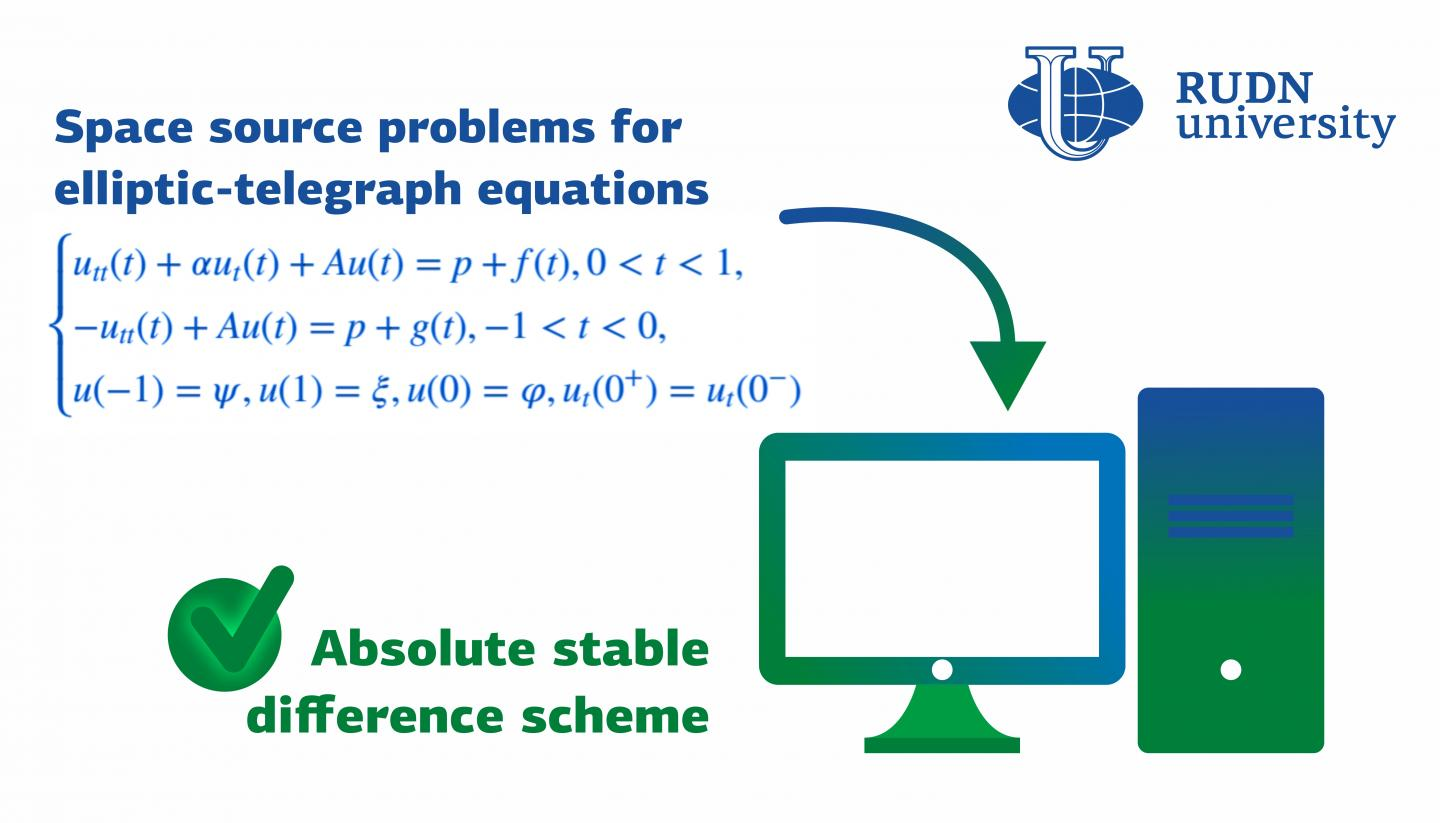News Release

# RUDN University mathematician suggested a scheme for solving telegraph equations

Peer-Reviewed Publication

RUDN Universityimage: A mathematician from RUDN University suggested a stable difference scheme for solving inverse problems for elliptic-telegraph and differential equations that are used to describe biological, physical, and sociological processes. view more  Credit: RUDN University

A mathematician from RUDN University suggested a stable difference scheme for solving inverse problems for elliptic-telegraph and differential equations that are used to describe biological, physical, and sociological processes. The results of the study were published in the Numerical Methods for Partial Differential Equations journal.

Elliptic equations are a class of differential equations in partial derivatives that are used, among other things, to model time-independent processes. Telegraph equations are presented in a nonstationary form. They were initially obtained for a telegraph communication line, but today they are also used to model the movement of insects, the flow of blood through veins, and the changes underwent by building materials. Moreover, they can be inversed, i.e. used to find a source of changes based on known process characteristics, for example, to identify a cause of material damage or to create an optic tomography image for the purposes of medical diagnostics. It is often difficult to obtain accurate solutions for problems like that, therefore, the initial problem is reduced to a system of simpler equations that provide an answer with a certain degree of approximation to the correct one. A mathematician from RUDN University suggested an algorithm to obtain inverse problem solutions for elliptic-telegraph equations using a computer.

"The more complex a modeled system, the more unknown parameters it contains, and the more difficult are the calculations. However, despite the complexity of the task, modern computers can be used to search for approximate solutions to differential equations. We aimed to obtain absolute stable difference schemes for the approximate solution of the space identification problem for the elliptic?telegraph equations. Our work could help further implement these methods into the modeling of various processes," said Prof. Allaberen Ashyralyev, a PhD in Physics and Mathematics from the Department of Higher Mathematics, RUDN University.

One way to obtain an approximate solution is to replace the initial problem with difference schemes. The studied area is turned into a grid with a given step size, and functions are replaced with nod values. The mathematician suggested a difference scheme and then studied it both analytically and numerically. The first method was used to confirm the absolute stability of the scheme, and the second (a numerical experiment, i.e. an equation that the scheme was applied to) - to support the results of the analysis. The scientist managed to demonstrate that the scheme was absolute stable and independent from the chosen calculation step size.

"Similar elliptic-telegraph equations are used to model biological systems, sociological phenomena, and engineering processes. An absolute stable difference scheme could help specialists better study these issues," added Prof. Allaberen Ashyralyev from RUDN University.

###

Disclaimer: AAAS and EurekAlert! are not responsible for the accuracy of news releases posted to EurekAlert! by contributing institutions or for the use of any information through the EurekAlert system.